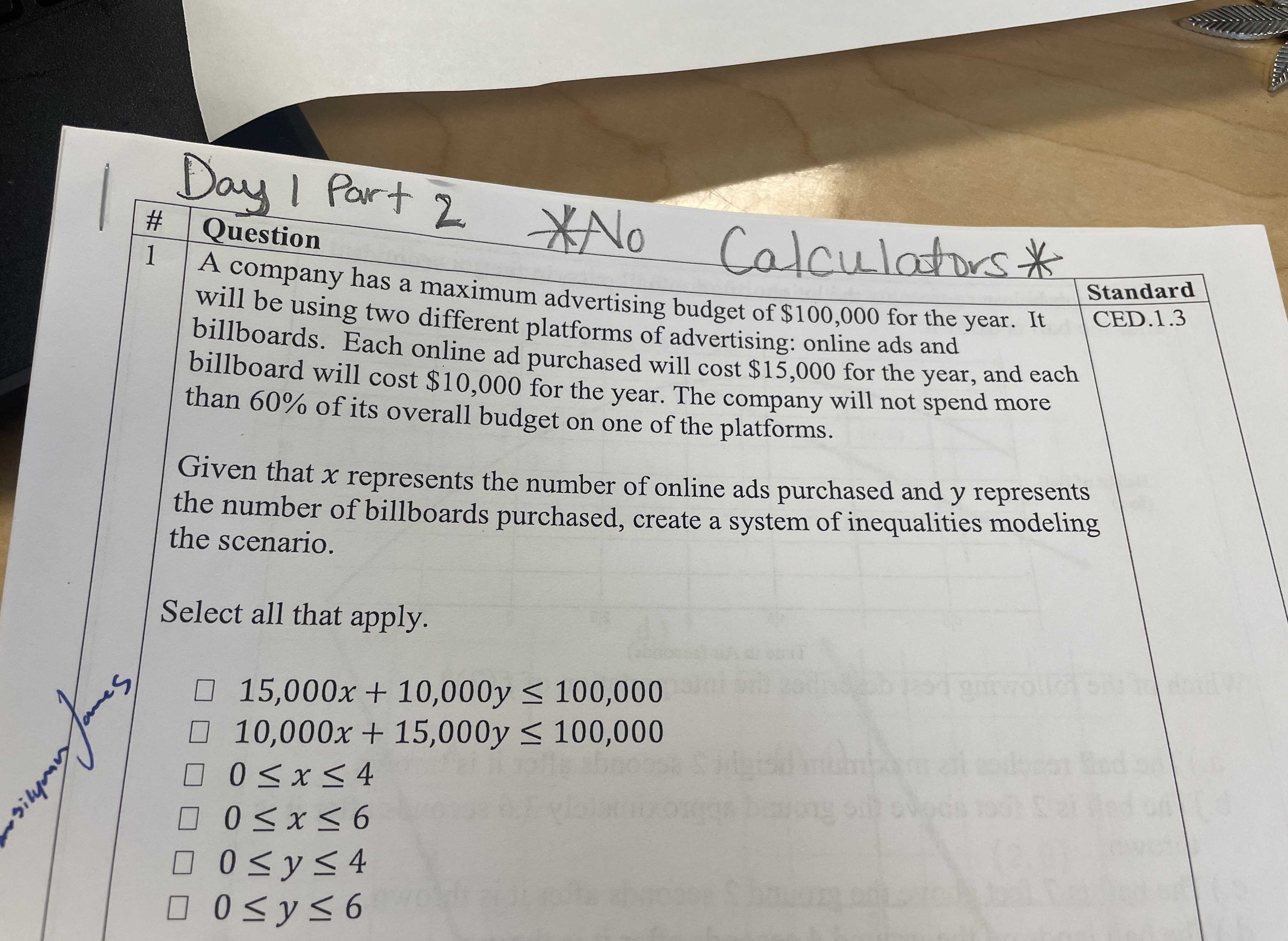### ¿Todavía tienes preguntas de matemáticas?

Pregunte a nuestros tutores expertos
Algebra
PreguntaA company has a maximum advertising budget of $$\ 100,000$$ for the year. It will be using two different platforms of advertising: online ads and billboards. Each online ad purchased will cost $$\ 15,000$$ for the year, and each billboard will cost $$\ 10,000$$ for the year. The company will not spend more than $$60 \%$$ of its overall budget on one of the platforms.

Given that $$x$$ represents the number of online ads purchased and $$y$$ represents the number of billboards purchased, create a system of inequalities modeling the scenario. Select all that apply.

$$\square 15,000 x + 10,000 y \leq 100,000$$

$$\square 10,000 x + 15,000 y \leq 100,000$$

$$\square 0 \leq x \leq 4$$

$$\square 0 \leq x \leq 6$$

$$\square 0 \leq y \leq 4$$

$$\square 0 \leq y \leq 6$$

15000x+10000y$$\le$$100000
0$$\le x\le$$6
$$0\le y\le 6$$